# AP SSC 10th Class Physics Solutions Chapter 7 Human Eye and Colourful World

AP State Board Syllabus AP SSC 10th Class Physics Solutions Chapter 7 Human Eye and Colourful World Textbook Questions and Answers.

## AP State Syllabus SSC 10th Class Physics Solutions 7th Lesson Human Eye and Colourful World

### 10th Class Physics 7th Lesson Human Eye and Colourful World Textbook Questions and Answers

Question 1.
What is the function of lens in human eye?
The eye lens forms a real image on retina then we can see the objects.

Question 2.
How does it help to see objects at long distances and short distances?
Eye lens can adjust itself in shape, so that it helps to see the objects at long and short distances.Question 3.
How is it possible to get the image at the same distance on the retina?
When the eye is focussed at distant object, the ciliary muscles are relaxed. So the focal length of eye lens adjusted itself which is equal to the distance of object from the retina. Then we can see the object clearly.
When the eye is focussed on a closer object the ciliary muscles adjust the focal length in such a way that the image is formed on retina and we see the object clearly.

Question 4.
Are we able to see all objects in front of our eye clearly?
Yes. By maintaining the 25 cm distance of the object from our eye, we can see the objects in front of our eye.

Question 5.
How do the lenses used in spectacles correct defects of vision?
To form image on retina.
To correct
myopia- Concave lens
hypermetropia i- Convex lens

Question 1.
How do you correct the eye defect Myopia? (AS1)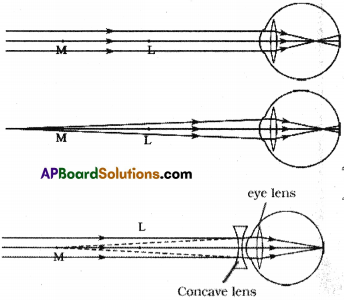• Some people cannot see objects at long distances but can see nearby objects clearly. This type of defect in vision is called ‘Myopia’ or ‘Near sightedness’.
• Myopia is corrected by using a con-cave lens of focal length equal to the distance of the far point F from the eye.
• This lens diverges the parallel rays from distant object as if they are coming from the far point.
• Finally the eye lens forms a clear im-age at the retina.

Question 2.
Explain the correction of the eye defect Hypermetropia. (AS1)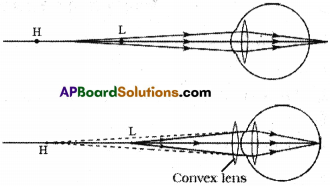• A person with hypermetropia can see distant objects clearly but cannot see objects at near distances. This is also known as ‘far sightedness’.
• Eye lens can form a clear image on the retina when any object is placed beyond near point.
• To correct the defect of hypermetropia, we need to use a lens which forms an image of an object beyond near point at H, when the object is between H and L. This is possible only when a double con¬vex lens is used.
• The image acts like an object for the eye lens. Hence final image due to eye is formed at retina.

Question 3.
How do you find experimentally the refractive index of material of a prism? (AS1)
(OR)
Write the experimental procedure in finding the refractive index of a prism.
(OR)
Which quantity will decide whether a given medium is denser or rarer? How do you find that quantity of prism experimentally?
Refractive index decides whether a medium is denser or rarer.
Aim :
To find the refractive index of a prism.

Materials required :
Glass prism, white chart of size 20 x 20 cm, pencil, pins, scale and protractor.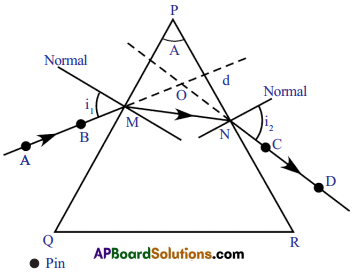Procedure :

1. Keep a prism on white chart.
2. Draw the triangular base of the prism with pencil.
3. Remove the prism.
4. The shape of the outline drawn prism is triangle and name its vertices as P, Q and R.
5. PQ and PR be the refracting surfaces.
6. Find the angle between PQ and PR. This is the angle of the prism (A) (or) Refracting angle.
7. Mark M on PQ and draw a perpendicular line to PQ at M.
8. Place the centre of the protractor at M, along the normal and mark an angle of 30° and then draw a line up to M. This line denotes incident ray. This angle is called angle of incidence.
9. Place the prism in its position (triangle) again.
10. Now fix two pins vertically on the line at points A and B .
11. See the images of pins through the 2nd refracting side (PR).
12. Fix another two pins at points C and D such that all the four pins appear to lie along the same line.
13. Remove the pins and prism, join the pin-holes. Draw the incident and emergent rays.
14. The angle between the normal and the emergent ray at N is the angle of emergence.
15. The line passing through the points A, B, M, N, C and D represents the path of light when it suffers refraction through prism.

The angle of deviation :

• Extend incident and emergent rays are intercept at a point ‘O’.
• The angle between these two rays is the angle of deviation (d).
• Note the emergent deviation angles for different values of i, in the given table.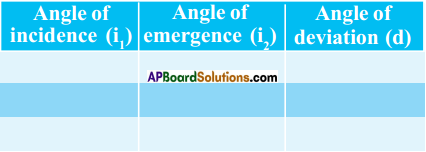• Draw the graph between angle of incidence on X – axis and the angle of deviation on Y – axis.
• We notice that the angle of deviation decreases first and then increases with increase of the angle of incidence.
• Mark points on a graph paper and join the points to obtain a graph (smooth curve).
• Draw a tangent line to the curve, which parallel to X-axis, at the lowest point of the graph.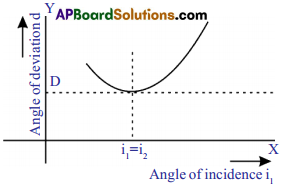• The point where this line cuts the Y- axis gives the angle of minimum deviation. It is denoted by D.
• From the graph, we notice that, at angle of minimum deviation, the angle of incidence is equal to the angle of emergence.
• By finding A and D we can find refractive index of prism by using formula .

Question 4.
Explain the formation of rainbow. (AS1)
(OR)
What is the natural spectrum occuring in sky? Explain the formation of that spectrum.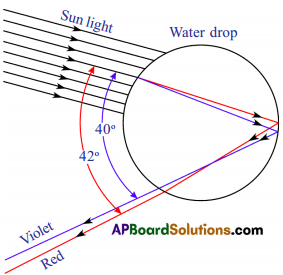• A rainbow is a natural spectrum of sunlight in the form of bows appearing in the sky when the sun shines on rain drops.
• It is combined result of reflection, refraction and dispersion of sunlight from water droplets, in atmosphere.
• It always forms in the direction opposite to the sun.
• To see a rainbow, the sun be must behind us and the water droplets falls in front of us.
• When a sunlight enters a spherical raindrop, it is refracted and dispersed. The different colours of light are bent in different angles.
• When different colours of light falls on the back inner surface of drop, it (Water drop) reflects (different colours of light) interwnally (total internal reflection).
• The water drops again refract the different colours, when it comes out from the raindrop.
• After leaving this different colours from the raindrop as rainbow, reach our eye. Thus, we see a rainbow.

Question 5.
Explain briefly the reason for the blue of the sky. (AS1)

• The reason for blue sky is due to the molecules N2 and O2.
• The size of these molecules are comparable to the wavelength of blue light.
• These molecules act as scattering centres for scattering of blue light.
• So scattering of blue light by molecules of N2 and O2 is responsible for blue of the sky.Question 6.
Explain two activities for the formation of artificial rainbow. (AS1)
(OR)
Give two activities for the formation of the artificial rainbow? And explain it.
(OR)
Suggest an experiment to create a rainbow in your classroom and explain the procedure.
Activity -1 :• Select a white coated wall on which the sun rays fdll.
• Stand in front of a wall in such a way that the sun rays fall on your back.
• Hold a tube through which water is flowing.
• Place your finger in the tube to obstruct the flow of water.
• Water comes out from small gaps between the tube and finger like a fountain.
• Observe the changes on wall while showering the water.

Activity – II : (Activity – 4)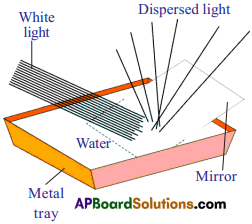• Take a metal tray and fill with water.
• Place a mirror in water such that it makes an angle to the water surface.
• Now focus white light on the mirror through the water.
• Keep a white card board sheet above the water surface.
• We may observe the colours VIBGYOR on the board.
• The splitting of white light into different colours (VIBGYOR) is called dispersion.
• So consider a white light is a collection of waves with different wavelengths.
• Violet has shortest wavelength, and red has longest wavelength.

Question 7.
Derive an expression for the refractive index of the material of a prism. (AS1)
Derivation of formula for refractive index of a prism :
PQ and PR are refracting surfaces of prism, i, is an angle of incidence, i2 is an angle of emergence, is an angle of refraction of first surface and r2 is an angle of incidence on second surface.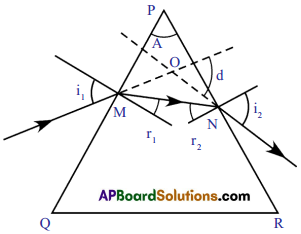1. From triangle OMN, we get
d = i1 – r1 + i2 – r2
∴ d = (i1 + i2)-(r1+ r2) ……… (1)
2. From triangle PMN, we have
∠A + (90° – r1) + (90° – r2) = 180°
⇒ r1 + r2 = A ……… (2)
3. From (1) and (2),
⇒ d = (i1 + i2) – A
⇒ A + d = i1 + i2
4. This is the relation between angle of incidence, angle of emergence, angle of deviation and angle of prism.

5. From Snell’s law, we know that
n1 sin i = n2 sin r

6. Let ‘n’ be the refractive index of the prism.
7. Using Snell’s law at M, refractive index of air n1 = 1; i = i1; n2 = n; r = r1.
⇒ sin i1 = n sin r1 …………. (4)
8. Similarly, at N, n1 = n; i = r2; n2 = 1; i1 = i2
⇒ n sin r2 = n sin i2 ………… (5)
9. We know that at the angle of minimum deviation position (D), i.e. i1 = i2
10. We will notice that MN is parallel to the base of prism QR.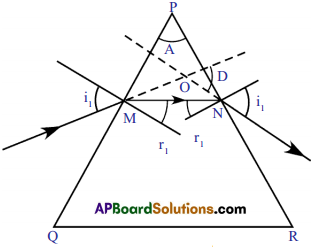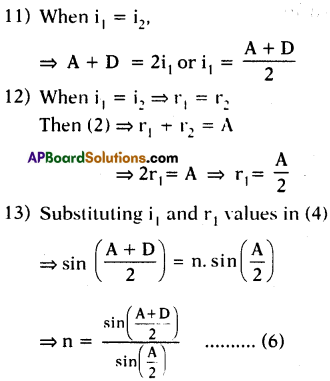14. This is the formula for the refractive index of the prism.

Question 8.
Light of wavelength λ1 enters a medium with refractive index n2 from a medium with refractive index n1 What is the wavelength of light in second medium? (AS1)
Wavelength of first medium =λ1
Refractive index of first medium = n1
Let the wavelength of second medium = λ2,
Refractive index of second medium = n2
Light enters from first medium to second medium = $$\Rightarrow \frac{\mathrm{n}_{2}}{\mathrm{n}_{1}}=\frac{\mathrm{c}_{1}}{\mathrm{c}_{2}}=\frac{\lambda_{1}}{\lambda_{2}}$$ (∵ υ = const)
$$\Rightarrow \lambda_{2}=\frac{\lambda_{1} \mathrm{n}_{1}}{\mathrm{n}_{2}}$$

Note:
For the below questions the following options are given. Choose the correct option by making hypothesis based on given assertion and reason. Give an explanation.
a) Both A and R are true and R is the correct explanation of A.
b) Both A and R are true and R is not the correct explanation of A.
c) A is true but R is false.
d) Both A and R are false.
e) A is false but R is true.Question 9.
Assertion (A) : The refractive index of a prism depends only on the kind of glass of which it is made of and the colour of light. (AS2)
Reason (R) : The refractive index of a prism depends on the refracting angle of the prism and the angle of minimum deviation.
(b) Both A and R are true and R is not the correct explanation of A.

Question 10.
Assertion (A) : Blue colour of sky appears due to scattering of light.
Reason (R) : Blue colour has shortest wavelength among all colours of white light. (AS2)
(C) A is true but R is false.

Question 11.
Suggest an experiment to produce a rainbow in your classroom and explain the procedure. (AS3)
Activity to produce a rainbow in classroom :

• Take a prism and place it on the table near a vertical white wall.
• Take a thin wooden plank, make a small hole in it and fix it vertically on the table.
• Place the prism between the wooden plank and wall.
• Place a white light source behind the hole of the wooden plank. Switch on the light.
• Adjust the height of the prism such that the light falls on one of the lateral surfaces.
• Observe the changes in the emerged ray of the prism.
• Adjust the prism by slightly rotating it till you get an image on the wall.
• We observe a band of different colours on the wall.
• These colours are nearly equal to the colours of the rainbow, i.e., VIBGYOR.Question 12.
Prisms are used in binoculars. Collect information why prisms are used in binoculars. (AS4)

• Binoculars consists of a pair of identical or mirror symmetrical telescope mounted side by side and aligned to point accurately in the same direction, allowing the viewer to use both eyes, when viewing distant objects.
• The size of binoculars is reduced by using prisms.
• We get good image with more brightness.
• Objective size and optical quality should be increased by using prisms in binoculars.

Question 13.
Incident ray on one of the face (AB) of prism and emergent ray from the face AC are given in figure. Complete the ray diagram. (AS5)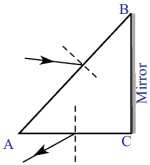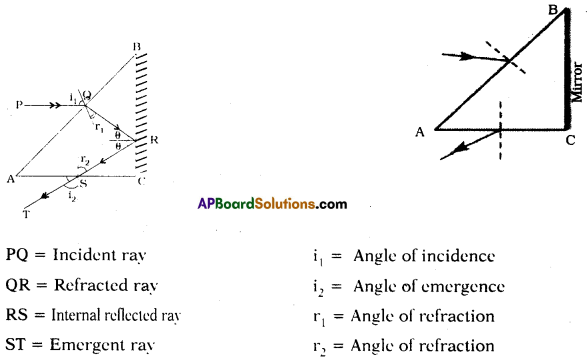Question 14.
How do you appreciate the role of molecules in the atmosphere for the blue colour of the sky? (AS6)
(OR)
How do you appreciate the nature of molecules, responsible for the blue of the sky?

• The sky appear blue due to atmospheric refraction and scattering of light through molecules.
• Molecules are scattering centres.
• The reason to blue sky is due to the molecules N2 and 02.
• The sizes of these molecules are comparable to the wavelength of blue light.
• In the absence of molecules there will be no scattering of sunlight and the sky will appear dark.
• We should appreciate the molecules which are scattering centres.

Question 15.
Eye is the only organ to visualise the colourful world around us. This is possible due to accommodation of eye lens. Prepare a six line stanza expressing your wonderful feelings. (AS6)
“Many people simply see
The world in black and white
But through my eyes the world’s alive
So colourful and bright
Don’t close your mind to the sights
That light up the night and day
There’s so much to see here on this earth
And not a rupee do you have to pay”
The most obvious things aren’t the ones
There is beauty in the unknown
with willing eyes and an open mind
The true wonders you will be shownQuestion 16.
How do you appreciate the working of ciliary muscles in the eye? (AS6)
(OR)
Which muscles are helpful in changing the focal length of eye lens? How do you appreciate those muscles?

• Ciliary muscles are helpful in changing focal length of eye lens.
• The ciliary muscles which attached with eye lens help to change the focal length of eye lens.
• When the eye is focussed on a distant object, these are relaxed. So the focal length of eye lens increases to its maximum value.
• The parallel rays coming into the eye are focussed on the retina then we can see the object clearly.
• When the eye is focussed on a nearer object the muscles are strained so the focal length of eye-lens decreases. The ciliary muscles adjust the focal length and the image is formed on retina then we can see the object.
• Their process of adjusting focal length of eve lens is called accomodation.
• So ciliary muscles must be appreciated for its accomodation of eye lens.

Question 17.
Why does the sky sometimes appear white? (AS7)
(OR)
The sky sometimes appears white. What is the reason behind it?

• Our atmosphere contains atoms and molecules of different sizes.
• According to their sizes, they are able to scatter different wavelengths of light.
• For example, the size of the water molecule is greater than the size of the N2 or O2.
• It acts as a scattering centre for light other frequencies which are lower than the frequency of blue light.
• On a hot day due to rise in the temperature, water vapour enters atmosphere which leads to abundant presence of water molecules in atmosphere. These water molecules scatter the colours of other frequencies (other than blue).
• All such colours of other frequencies reach our eye and white colour is appeared to us.

Question 18.
Glass is known to be transparent material. But ground glass is opaque and white in colour. Why? (AS7)

• Ground glass is glass whose surface has been ground that produces a flat but rough finish.
• Ground glass has the effect of rendering the glass translucent by scattering of light during transmission thus blurring visibility while still transmitting light.
• To get more permanent frost, the glass may be ground by rubbing with some gritty substance.Question 19.
If a white sheet of paper is stained with oil, the paper turns transparent. Why? (AS7)
(OR)
What is the reason behind the paper turns transparent when it is immersed in oil?

• The paper fibres have higher index of refraction probably much greater than 1.5.
• The oil or fat also has a high index of refraction so that it nearly matches the index of refraction of the paper fibres and it reduces the scattering significantly.
• The fat adhering to the cellulose fibers lowers the index of refraction of the cellulose and also fills in air voids, so that visible light passes through the bag with significantly less scattering.
• The oil connects the fibres in the paper with a liquid which can transmit by refraction (rather than scatter) light that falls upon it. As a result, the paper stained with oil is turned transparent.

Question 20.
A light ray falls on one of the faces of a prism at an angle 40° so that it suffers angle of minimum deviation of 30°. Find the angle of prism and angle of refraction at the given surface. (AS7)
Given that, incident ray on one of the prisms (i1) = 40°
Angle of minimum deviation (D) = 30°
Angle of prism (A) = ?
A+D = 2i1
⇒ A = 2i1 – D
⇒ A = 2(40°) -30°
= 80° – 30°
= 50°
∴ Angle of prims (A) = 50°
Angle of refraction (r1) = ?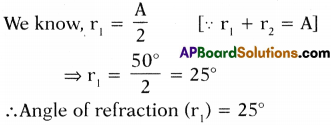Question 21.
The focal length of a lens suggested to a person with Hypermetropia is 100 cm. Find the distance of near point and power of the lens. (AS7)
i) The distance of near point :
If the distance of near point is ‘d’ and focal length is ‘f’ then the relation between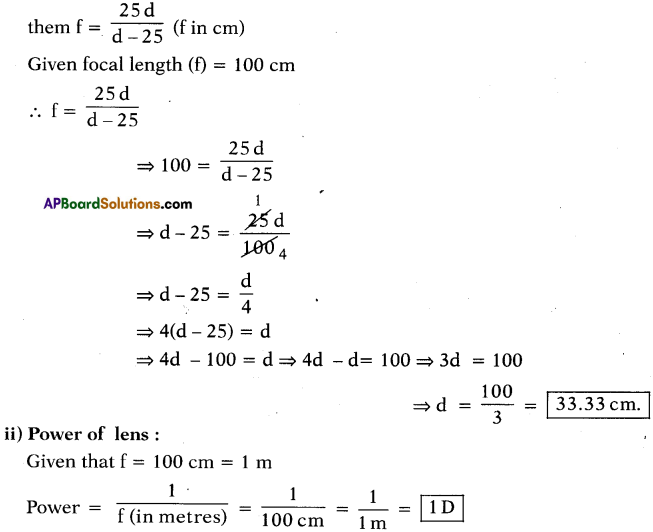Question 22.
A person is viewing an extended object. If a converging lens is placed in front of his eye, will he feel that the size of object has increased? Why? (AS7)

• A simple magnifier allows us to put the object closer to the eye than we could normally focus and forms an enlarged virtual images.
• The principle behind this is angular magnification.
• The magnification is Ma = $$\frac{25}{f}$$ for the close focus point, but since that causes eye strain, it is usually desirable to put the images at infinity giving Ma = $$\frac{25}{f}$$
• So he fees the size of object is increased. The reason is mentioned.
• Angular magnification: ‘The ratio of the angle substended at the eye by the image formed by an optical instrument to the angle substended at the eye by the object being viewed.”

Fill In The Blanks

1. The value of least distance of distinct vision is about ……………………… .
2. The distance between the eye lens and retina is about ……………………… .
3. The maximum focal length of the eye lens is about ……………………… .
4. The eye lens can change its focal length due to working of ……………………… muscles.
5. The power of lens is ID then focal length is ……………………… .
6. Myopia can be corrected by using ……………………… lens.
7. Hypermetropia can be corrected by using ……………………… lens.
8. In minimum deviation position of prism, the angle of incidence is equal to angle of ……………………… .
9. The splitting of white light into different colours (VIBGYOR) is called ……………………… .
10. During refraction of light, the character of light which does not change is ……………………… .

1. 25 cm
2. 2.5 cm
3. 2.5 cm
4. ciliary
5. 100 cm
6. bi-concave
7. bi-convex
8. emergence
9. dispersion of light
10. frequency

Multiple Choice Questions

1. The size of an object as perceived by an eye depends primarily on
A) actual size of the object
B) distance of the object from the eye
C) aperture of the pupil
D) size if the image formed on the retina
B) distance of the object from the eye

2. When objects at different distances are seen by the eye which of the following remains constant?
A) focal length of eye-lens
B) object distance from eye-lens
C) the radii of curvature of eye-lens
D) image distance from eye-lens
D) image distance from eye-lens3. During refraction, …………….. will not change.
A) wavelength
B) frequency
C) speed of light
D) all the above
B) frequency

4. A ray of light falls on one of the lateral surfaces of an equilateral glass prism placed on the horizontal surface of a table as shown in figure. For minimum deviation of ray, which of the following is true?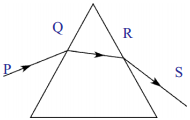A) PQ is horizontal
B) ‘QR’ is horizontal
C) ‘RS’ is horizontal
D) Either ‘PQ’ or ‘RS’ is horizontal
B) ‘QR’ is horizontal

5. Far point of a person is 5 m. In order that he has normal vision what ki nd of spectacles should he use?
A) concave lens with focal length 5 m
B) concave lens with focal length 10 m
C) convex lens with focal length 5 m
D) convex lens with focal length 2.5 mm
A) concave lens with focal length 5 m

6. The process of re-emission of absorbed light in all directions with different intensi¬ties by the atom or molecule is called ……………
A) scattering of light
B) dispersion of light
C) reflection of light
D) refraction of light
A) scattering of light

### 10th Class Physics 7th Lesson Human Eye and Colourful World InText Questions and Answers

10th Class Physics Textbook Page No. 104

Question 1.
Can you imagine the shape of rainbow when observed during travel in an airplane?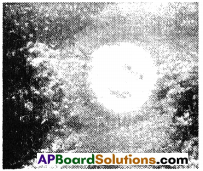The shape of rainbow, when observed during travel in an airplane is circular as shown in the following figure.

10th Class Physics Textbook Page No. 88

Question 2.
Why do the values of least distance of distinct vision and angle of vision change with person and age?

• The ciliary muscle helps the eye lens to change its focal length by changing radii of curvature of eye lens.
• When the eye is focussed on a distant object, the ciliary muscles are relaxed so that the focal length of eye lens has its maximum value which is equal to its distance from the retina.
• The working of ciliary muscle in eye changes from person to person.
• So, the values of least distance of distinct vision and angle of vision changes with person and age.

10th Class Physics Textbook Page No. 89

Question 3.
How can we get same image distance for various positions of objects?
For different positions of object, the image distance remains constant only when focal length of lens changes.Question 4.
Can you answer above question using concepts of refraction through lenses?
The focal length of a lens depends on the material by which it has been made and radii of curvatures of lens. We need to change focal length of eye lens to get same image distance for various positions of object.

10th Class Physics Textbook Page No. 90

Question 5.
How does eye lens change its focal length?
(OR)
What is the role of ciliary muscles in the eye ? Write the answer in one or two sentences only.
The ciliary muscles to which eye lens is attached help the eye lens to change its focal length by changing the radii of curvature of eye lens.

Question 6.
How does this change (eye lens changes its focal lengths) take place in eye ball?
When the eye is focussed on a distant object the ciliary muscles are relaxed. So the focal length of eye lens has its maximum value which is equal to its distance from the retina. The parallel rays coming into the eye are then focussed on to the retina and we see the object clearly.Question 7.
Does eye lens form a real image or virtual image?
The eye lens forms a real and inverted image of an object on the retina.

Question 8.
How does the image formed on retina help us to perceive the object without change in its shape, size and colour?

• The eye lens forms a real and inverted image of an object on the retina.
• This retina is a delicate membrane, which contains large number of receptors (125 million) called ‘rods’ and ‘cones’.
• They receive the light signals and identify the colour, and the intensity of light.
• These signals are transmitted to the brain through optic-nerve fibres.
• The brain interprets these signals and finally processes the information so that we perceive the object in terms of its shape, size and colour.

Question 9.
Is there any limit to change of focal length of eye lens?
Yes. When the object is at infinity, the parallel rays from the object falling on the eye lens are refracted. They form a point-sized image on retina. In this situation, eye lens has a maximum focal length.

Question 10.
What are the maximum and minimum focal lengths of the eye lens?
Maximum focal length is 2.5 cm and minimum focal length is 2.27 cm.Question 11.
How can we find the maximum and minimum focal lengths of the eye lens?
(OR)
Calculate the maximum and minimum focal lengths of the eye lens.
a) When the object is at infinity,
u = – ∞ ; v = 2.5 cm (image distance which is equal to distance between eye lens and retina)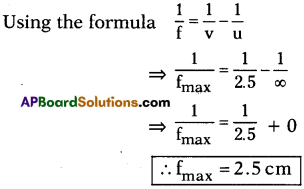b) Object at a distance of 25 cm from eye. In this case, eye has minimum focal length.
Here u = -25 cm ; v 2.5 cm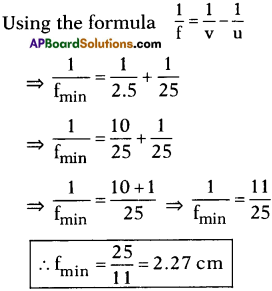10th Class Physics Textbook Page No. 91

Question 12.
What happens if eye lens is not able to adjust its focal length?
In this case the person cannot see the object clearly and comfortably.Question 13.
What happens if the focal length of eye lens is beyond the range of 2.5 cm to 2.27 cm?
The vision (image) becomes blurred due to defects of eye lens.

10th Class Physics Textbook Page No. 92

Question 14.
What can we do to correct myopia?
To correct myopia, we use concave lens.

10th Class Physics Textbook Page No. 93

Question 15.
How can you decide the focal length of the lens to be used to correct myopia?
Assume that the object distance (u) is infinity and image distance (v) is equal to distance of far point.
u = – ∞ ; v = distance of far point = – D
Let ‘f be the focal length of bi-concave lens.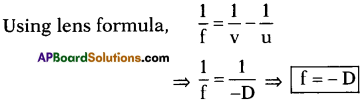Here ‘f is negative showing that it is a concave lens.

Question 16.
What happens when the eye has a minimum focal length greater than 2.27 cm?
In this case, the rays coming from the nearby object after refraction at eye lens, forms image beyond the retina.

10th Class Physics Textbook Page No. 94

Question 17.
How can you decide the focal length of convex lens to be used?
Here object distance (u) = -25 cm
Image distance (v) = – d (distance of near point)
Let ‘f be the focal length of bi-convex lens.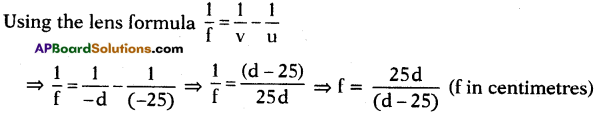If d > 25 cm, then ‘f’ becomes positive then we need to use bi-convex lens to correct hypermetropia.

10th Class Physics Textbook Page No. 95

Question 18.
Have you ever observed details in the prescription?
A prescription that contains some information regarding type of lens to be used to correct vision.

Question 19.
What does it (“my sight is increased or decreased”) mean?
Usually doctors, after testing the defects of vision prescribe corrective lenses indicating
their power which determines the type of lens to be used and its focal length.Question 20.
What do you mean by power of lens ? flftorH)
The reciprocal of focal length is called power of lens.

Question 21.
Doctor advised to use 2D lens. What is its focal length?
Given power of lens P = 2D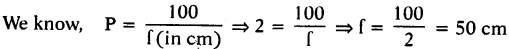∴ Focal length of lens (f) = 50 cm.

10th Class Physics Textbook Page No. 96

Question 22.
How could the white light of the sun give us various colours of the rainbow?
Due to reflection, refraction and dispersion of sunlight.

Question 23.
What happens to a light ray when it passes through a transparent medium bounded by plane surfaces which are inclined to each other?
When light incident on one of the plane surfaces and emerges from the other.Question 24.
What is a prism?
A prism is a transparent medium separated from the surrounding medium by consisting two refracting plane surfaces which are inclined.

10th Class Physics Textbook Page No. 97

Question 25.
What is the shape of the outline drawn for a prism?
The outline drawn for a prism is in a triangle shape.

10th Class Physics Textbook Page No. 98

Question 26.
How do you find the angle of deviation?
The angle between the extended incident and emergent rays is called angle of deviation.
(OR)
Extend both incident and emergent rays till they meet at a point ‘O’. Measure the angle between these two rays. This is the angle of deviation.

Question 27.
What do you notice from the angle of deviation?
The angle of deviation decreases first and then increases with increase in the angle of incidence.

Question 28.
Can you draw a graph between angle of incidence and angle of deviation?Yes, we can draw the graph between angle of incidence and angle of deviation.

Question 29.
From the graph, can you find the minimum of the angle of deviation?
Yes, we can. Draw a tangent line to the curve, parallel to X – axis, at the lowest point of the graph. The point where this line cuts Y – axis gives the angle of minimum deviation.

Question 30.
Is there any relation between the angle of incidence (i) angle of emergence (r) and piangle of deviation (d)?
(i1 + i2)= A + D
i + r = A + D

10th Class Physics Textbook Page No. 101

Question 31.
In activity-3,we noticed that light has chosen different paths. Does this mean that the refractive index of the prism varies from colour to colour?
Yes, refractive index of the prism varies from colour to colour.

Question 32.
Is the speed of light of each colour different?
In vacuum – No, the speed of each colour is constant.
In medium – Speed is different for different colours.Question 33.
Can you guess now, why light splits into different colours when it passes through a prism?
Due to dispersion of light and different wavelength of colours in medium.

10th Class Physics Textbook Page No. 102

Question 34.
Does it (light passing through a prism) split into more colours? Why?
We know the frequency of light is the properly of the source and it is equal to number of waves leaving the source per second. This cannot be changed by any medium. Hence frequency doesn’t change due to refraction. The coloured light passing through any transparent medium retains its colour.

Question 35.
Can you give an example in nature, where you observe colours as seen in activity 3?
Yes, in rainbow. It is a good example of dispersion of light.Question 36.
When do you see a rainbow in the sky?
Dut to the refraction, reflection and dispersion of sunlight, when the sunlight passes through the rain drops, we can see the rainbow in the sky.

Question 37.
Can we create a rainbow artificially?
Yes, we can create a rainbow artificially.

10th Class Physics Textbook Page No. 104

Question 38.
Why does the light dispersed by the raindrops appear as a bow?

• A rainbow is not the flat two dimensional arc as it appears to us.
• The rainbow we see is actually a three dimensional cone with the tip of our eye.
• All the drops that disperse the light towards us lie in the shape of the cone – a cone of different layers.
• The drops that disperse red colour to our eye are on the outermost layer of the cone, similarly, the drops that disperse orange colour to our eye are on the layer of the cone beneath the red colour cone.
• In this way the cone responsible for yellow lies beneath orange and so on it till the violet colour cone becomes the innermost cone.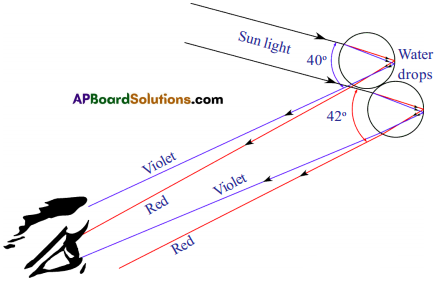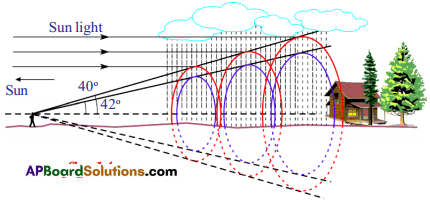Question 39.
Why is the sky blue?
A clear cloudless day – time sky is blue because molecules in the air scatter blue light from the sun more than thev scatter red liuht.

Question 40.
What is scattering?
A. Atoms or molecules which are exposed to absorb light energy and emit some part of the light energy in different directions is called scattering of light.

10th Class Physics Textbook Page No. 106

Question 41.
Why is that the sky appears white sometimes when you view it in certain direction on hot days?

• On a hot day, due to rise in the temperature, water vapour enters atmosphere which leads to abundant presence of water molecules in atmosphere.
• These water molecules scatter the colours of other frequencies (other than blue).
• All such colours of other frequencies reach our eye and the sky appears white.

Question 42.
Do you know the reasons for appearance the red colour of sun during sunrise and at sunset?
(OR)
Sun appears red in colour during sunrise and sunset. Give reason.
(OR)
Why does sky appear red at Sunshine and Sunset?

• The light rays from the sun travel more distance in atmosphere to reach our eye in morning and evening times.
• During sunrise and sunset except red light all colours scatter more and vanish before they reach us.
• Since scattering of red light is very less, it reaches us.
• As a result sun appears red in colour during sunrise and sunset.Question 43.
Can you guess the reason why sun does not appear red during noon hours?
During noon hours, the distance to be travelled by the sun rays in atmosphere is less than when compared to morning and evening hours. Therefore all colours reach our eye without scattering. Hence the sunlight appears white in noon hours.

10th Class Physics 7th Lesson Human Eye and Colourful World Activities

Activity – 1

Question 1.
How do you find least distance of distinct vision?
(OR)
What is the least distance a person can see an object comfortably and distinctly known as ? Write an activity to find that (least, distance of distinct vision) distance.

• The least distance a person can see an object comfortably and distinctly is known as least distance of distinct vision.
• Hold the textbook at certain distance with your hands.
• Try to read the contents on the page.
• Gradually move the book towards eye, till it reaches very close to your eyes.
• You may see that printed letters on the page appear blurred or you feel strain to read.
• Now move the book backwards to a position where you can see clear printed letters without strain.
• Note down its value.
• Repeat this activity with other friends and note down the distances for distinct vision in each case.
• Find the average of all these distances of clear vision.
• You will notice that to see an object comfortably and distinctly, you must hold it at a distance about 25 cm from your eyes.
• This 25 cm distance is called least distance of distinct vision.
• This value varies from person to person and with age.

Activity – 2

Question 2.
How can you find angle of vision?
(OR)
What is maximun angle a person is able to see whole object? Write an activity to find that angle.• The maximum angle, at which we are able to see the whole object is called angle of vision.
• Arrange a retort stand.
• Collect a few wooden sticks (or) PVC pipes that are used for electric wiring.
• Prepare sticks or pipes of 20 cm, 30 cm, 35 cm, 40 cm, 50 cm from them.
• Keep the retort stand on a table and stand near the table such that vour head is beside the vertical stand.
• Adjust the clamp on horizontal rod and fix at a distance of 25 cm from the eyes.
• Ask one of your friends to fix a wooden stick of 30 cm height to the clamp in vertical position.
• Now keeping your vision parallel to horizontal rod of the stand, try to see the top and bottom of wooden stick kept in vertical position.
• If you are not able to see both ends of stick at this distance (25 cm), adjust the vertical stick on the horizontal rod till you are able to see both ends of the stick at nearest possible distance from your eye.
• Fix the clamp to the vertical stick at this position.
• Without changing the position of the clamp on horizontal rod, replace this stick of 30 cm length.
• Try to see the top and bottom of the stick simultaneously without any change in the position of eye.
• Try the same activity with various lengths of the sticks.
• You can see the whole object AB which is at a distance of 25 cm (least distance of clear vision) because the rays coming from the ends A and B of the object will enter the eye.
• Similarly we can also see complete object CD with eye as explained above.
• Let us assume that AB is moving closer to eye to a position A B .
• You notice that you will be able to see only the part (EF) of the object A B because the rays coming from E and F enter your eye.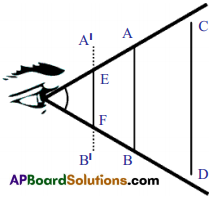• The rays coming from A and B cannot enter your eye.
• The rays coming from the extreme ends of an object forms an angle at the eye.
• If this angle is below 60°, we can see whole object.
• If this angle is above 60°, then we see only the part of the object.
• This maximum angle, at which we are able to see the whole object is called angle of vision.
• The angle of vision for a healthy human being is about 60°.
• It varies from person to person and with age.

Activity – 3

Question 3.
Describe an activity for dispersion of light.
(OR)
What is the name given to process when white light passes through a prism it splits into different colours ? Explain the process with an activity.

• The splitting of white light into different colours is called despersion of light.
• Do this experiment in the dark room.
• Take a prism and place it on the table near a vertical white wall.
• Take a thin wooden plank.
• Make a small hole in it and fix it vertically on the table.
• Place the prism between the wooden plank and wall.
• Place a white light source behind the hole of wooden plank.
• Switch on the light.
• The rays coming out of the hole of plank become a narrow beam of light.
• Adjust the height of the prism such that the light falls on one of the lateral surfaces.
• Observe the changes in emerged rays of the prism.
• Adjust the prism by slightly rotating it till you get an image on the wall.
• You will observe that white light is splitting into certain different colours.

Activity – 6

Question 4.
Describe an experiment for scattering of light.
(OR)
What is the principle involved in blue of the sky ? Explain the principle with an experiment?

• Take the solution of sodium-thio-Sulphate (hypo) with sulphuric acid in a glass beaker.
• Place the beaker in which reaction is taking place in an open place where abundant sunlight is available.
• Watch the formation of grains of sulphur and observe changes in beaker.
• You will notice that sulphur precipitates as the reaction is in progress.
• At the beginning, the grains of sulphur are smaller in size as the reaction progresses, their size increases due to precipitation.
• Sulphur grains appear blue in colour at beginning and slowly their colour becomes white as their size increases.
• The reason for this is scattering of light.
• At the beginning, the size of grains is small and almost comparable to the wavelength of blue light.
• Hence they appear blue in the beginning.
• As the size of grains increases, their size becomes comparable to the wavelengths of other colours.
• As a result of this, they act as scattering centres for other colours.
• The combination of all these colours appears as white.